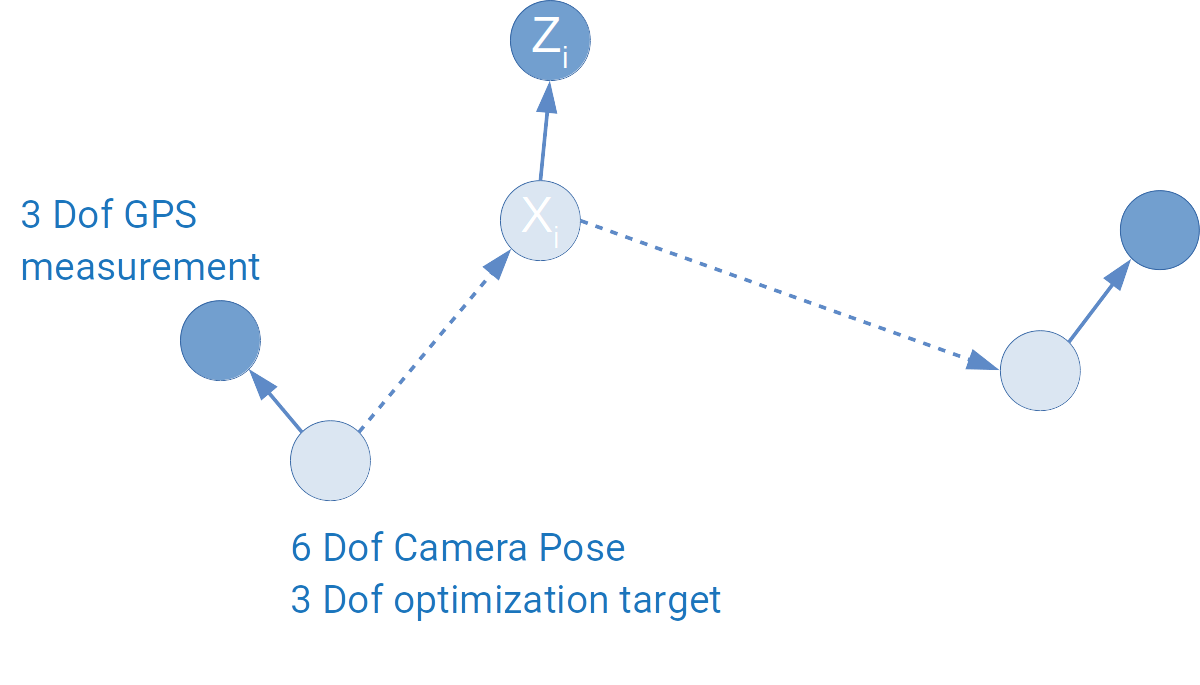# Graph Optimization 4 - g2o introduction - GPS odometry

In graph optimization 1 to 3, the math is introduced. Now we can make some hands on practice on programming. The most famous used graph optimization library is g2o due to its good performance in ORB-SLAM. g2o also has its well known drawback - not well commented, not easy to understand. What’s more, most of the tutorials are based on the original sample examples, when you want to make your own vertex or edge, you will again be lost.

This introduction will be based on an easy to understand graph optimization problem with a customized edge implementation.

# Modelling the GPS based odometry

## Model as graph

The problem is quite easy: we have a vehicle moving around, we use a GPS to measure its 3D absolute positions, by making some rough guesses as initialization, we want to estimate the vehicle’s position based on the GPS’ measurements.

In a SLAM system, we usually want to fuse different sensors, what’s discussed most are fusing camera and IMU, which is also the problem setup for g2o examples. Fusing GPS information is rarely touched, actually GPS sensor fusion is easier to understand. It can be modeled as the following diagram:# Graph Optimization 3 - Optimization Update

From the last article, we get the following negative log likelihood function as our optimization target:

$F(x)=\sum_{ij}{e_{ij}(x)^T\Omega_{ij}e_{ij}(x)}$

The optimization problem turns to be:

$x^*=\arg\min_xF(x)$

This article will explain how can this optimization problem be solved using Gauss-Newton method.

# Graph Optimization 2 - Modelling Optimization Target

Usually, all graph optimization papers or tutorials start from raising the optimization problem: minimizing the following function:

$F(x)=\sum_{ij}{e_{ij}(x)^T\Omega_{ij}e_{ij}(x)}$

This article will explain where this optimization comes from and why it is related to Gaussian noise.

## Maximum Likelihood Estimation with Gaussian noise

The probabilistic modeling of graph optimization is based on the Maximum Likelihood Estimation(MLE) algorithm. (More information on Chapter 6 of Xiang Gao’s book.).

The case we use for this article will also be the one we used in the last article: